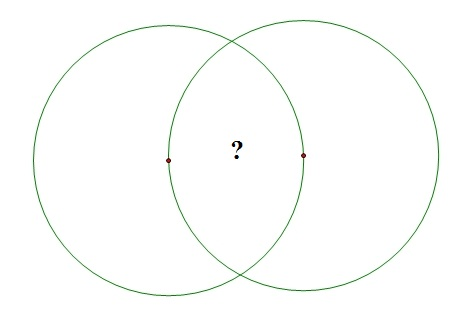Congruent Circles Intersection

Geometry Level 3The above diagram shows 2 congruent circles of radius 6, and each circle passes through the center of the other circle.

To the nearest whole number, what is the area common to the two circles?

×